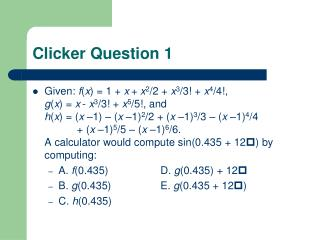DownloadDownload PresentationClicker Question 1

# Clicker Question 1

Download Presentation## Clicker Question 1

- - - - - - - - - - - - - - - - - - - - - - - - - - - E N D - - - - - - - - - - - - - - - - - - - - - - - - - - -
##### Presentation Transcript

1. Clicker Question 1 • Given: f(x) = 1 + x+ x2/2 + x3/3! + x4/4!,g(x) = x- x3/3! + x5/5!, andh(x) = (x –1) – (x –1)2/2 + (x –1)3/3 – (x –1)4/4 + (x –1)5/5 – (x –1)6/6.A calculator would compute sin(0.435 + 12) by computing: • A. f(0.435) D. g(0.435) + 12 • B. g(0.435) E. g(0.435 + 12) • C. h(0.435)

2. Clicker Question 2 • Given: f(x) = 1 + x+ x2/2 + x3/3! + x4/4!,g(x) = x- x3/3! + x5/5!, andh(x) = (x –1) – (x –1)2/2 + (x –1)3/3 – (x –1)4/4 + (x –1)5/5 – (x –1)6/6.A calculator would compute e6.435by computing: • A. f(0.435) D. 6(2.7183) + f(0.435) • B. g(0.435) E. (2.7183)6f(0.435) • C. h(0.435)

3. Clicker Question 3 • Given: f(x) = 1 + x+ x2/2 + x3/3! + x4/4!,g(x) = x- x3/3! + x5/5!, andh(x) = (x –1) – (x –1)2/2 + (x –1)3/3 – (x –1)4/4 + (x –1)5/5 – (x –1)6/6, and ln(10)  2.3026.A calculator would compute ln(6.435) by computing: • A. f(6.435) D. 2.3026 + h(0.6435) • B. g(6.435) E. 2.3026 h(0.6435) • C. h(6.435)

4. Assignment for Monday • Work on Hand-in #5 (due Monday at 4 pm). • On Monday we’ll tie up loose ends, review, return the clickers, and have evaluations of the course.